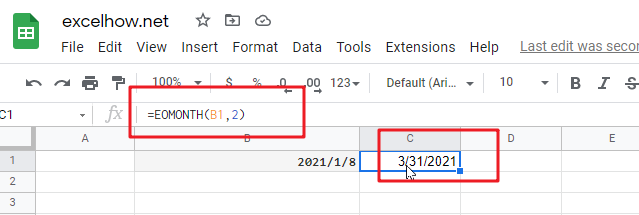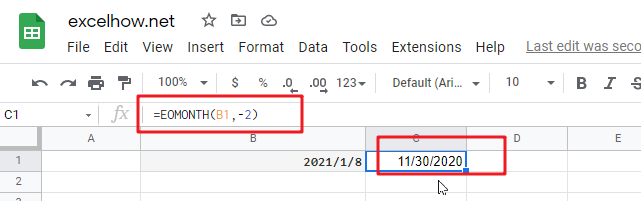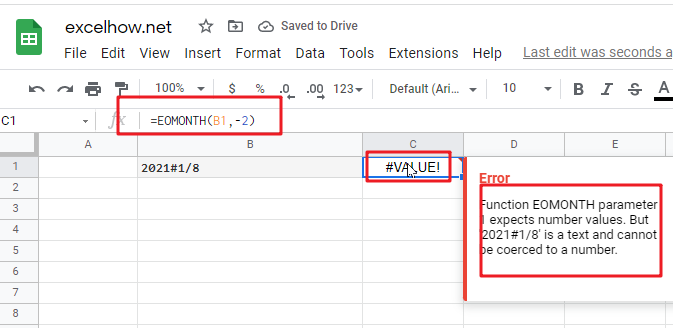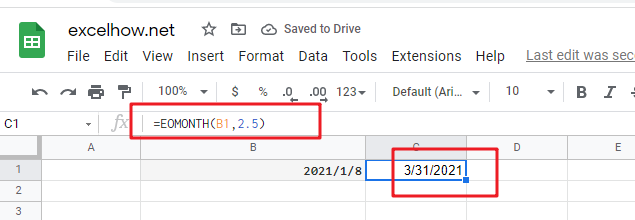# ExcelHow

This post will guide you how to use Google Sheets EOMONTH function with syntax and examples.

## Description

The Google Sheets EOMONTH returns a date representing the last day of the month, n months in the future or past.

The EOMONTH function can be used to get a date that representing the last day of a month which falls a specified number of months before or after date in google sheets. The purpose of this function is to get last day of month or n months in the future or past and its returned values is a last day of month date.

The EOMONTH function is a build-in function in Google Sheets and it is categorized as a Date function.

## Syntax

The syntax of the EOMONTH function is as below:

=EOMONTH (start_date, months)

Where the EOMONTH function arguments are:

• Start_date -This is a required argument. A date that represents the start date.
• months – This is a required argument. the number of months before or after start_date. if you provide a positive number for months, it represents the future date; if it is a negative number, then it represents a past date.

Notes:

• google sheets stores dates as a serial number, it indicated the number of days since January 1,1900. and the date January 1,1900 is serial number 1.
• if start_date is not a valid date, the EOMONTH function will return the #VALUE! error.
• if months is not an integer, it is truncated.
• If the start_date has a fractional time component, it will be removed.
• If the start_date argument contains a decimal value, it will be removed.
• if you want to change any date n months into the future or past date, you can use the EDATE function.

## Google Sheets EOMONTH Function Examples

The below examples will show you how to use google sheets EOMONTH Function to return the serial number.
1# set a positive number for months argument in the EOMONTH function

`=EOMONTH(B1,2)`2# set a negative number for months argument in the EOMONTH function, using the following formula:

`=EOMONTH(B1,-2)`3# set an invalid date for start_date argument in the EOMONTH function, type the following formula:

`=EOMONTH(B1,-2)`4# set a value that is not an integer for months arguments, using the following formula:

`=EOMONTH(B1,2)`